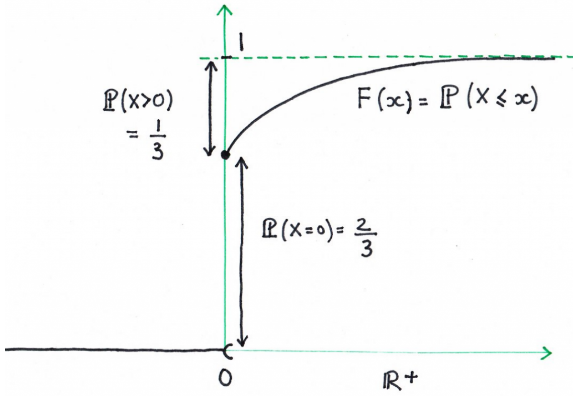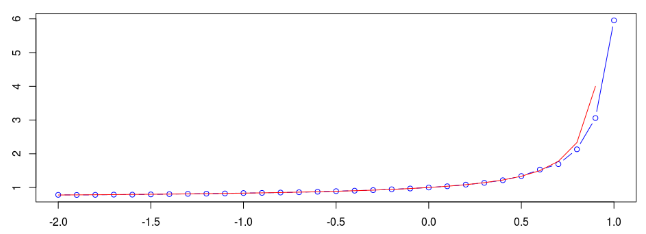# R语言蒙特卡洛计算和快速傅立叶变换计算矩生成函数

• 如果 ​ 是离散的

• 如果 ​ （绝对）连续，

​ 是的密度 ​。这里， ​ 显然不是离散变量。但是是连续的。需要绘制该分布函数以查看， ​， 对所有$latex i\hbar\frac{\partial}{\partial }\left|\Psi(t)\right>=H\left|\Psi(t)\right>$

（因为它是一个实值常量），在这里 ​。

• 蒙特卡洛计算

> F=function(x) ifelse(x<0,0,1-exp(-x)/3)
> Finv=function(u) uniroot(function(x) F(x)-u,c(-1e-9,1e4))$root 或（以避免不连续的问题） > Finv=function(u) ifelse(3*u>1,0,uniroot(function(x) + F(x)-u,c(-1e-9,1e4))$root))


> plot(u,v,type="b",col='blue')
> lines(u,Mtheo(u),col="red")> M(3)
 5748134

> mean(rcauchy(1000000))
 0.006069028

• 生成函数

可以为某些随机矢量在更高维度上定义一个矩生成函数 ​，

​如果要导出给定分布的矩，则一些矩生成函数很有趣。另一个有趣的特征是，在某些情况下，此矩生成函数（在某些条件下）完全表征了随机变量的分布。 ​，
​ 对所有人 ​， 然后 ​。

• 快速傅立叶变换

• 特征函数和精算科学

​ 和 ​。策略是分散损失金额，

99.5％分位数

> sum(cumsum(f)<.995)


> print(X[1:5])
 75.51818 118.16428 14.57067 13.97953 43.60686

      shape         rate
1.309020256   0.013090411
(0.117430137) (0.001419982)


> alpha
 1.308995
> beta
 0.01309016

> lambda <- 100

> set.seed(1)
> quantile(rcpd4(1e6),.995)
99.5%
13651.64

> uniroot()\$root
 13654.43

> sum(cumsum(f)<.995)
 13654

> system.time
user      system     elapsed
2.453       0.106       2.611
> system.time
user      system     elapsed
0.041       0.012       0.361
> system.time
user      system     elapsed
0.527       0.020       0.560

Kaizong Ye拓端研究室（TRL）的研究员。

​非常感谢您阅读本文，如需帮助请联系我们！QQ在线咨询

15121130882

0571-63341498

## 关注有关新文章的微信公众号

This will close in 0 seconds